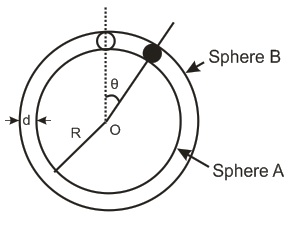# Newton's Ball!A spherical ball of mass $m$ is kept at the highest point in the space between two fixed concentric spheres $A$ and $B$ . The smaller sphere $A$ has a radius $R$ and the space between the two spheres has a width $d$. The ball has a diameter very slightly less than $d$ . All surfaces are frictionless. The ball is given a gentle push towards the right . The angle made by the radius vector of the ball with the upward vertical is $\theta$. What is the total normal reaction force exerted by the spheres on the ball in terms of angle $\theta$?

×